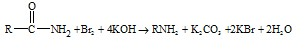Q

# Please help! In the Hofmann bromamide degradation reaction, the number of moles of NaOH and Br2 used per mole of amine produced are :

In the Hofmann bromamide degradation reaction, the number of moles of NaOH and Br2 used per mole of amine produced are :

• Option 1)

One mole of NaOH and one mole of Br2 .

• Option 2)

Four moles of NaOH and two moles of Br2 .

• Option 3)

Two moles of NaOH and two moles of Br2 .

• Option 4)

Four moles of NaOH and one mole of Br2 .

205 Views

As we learnt in

Amines ( only primary ) can also be prepared by Hofmann degradation. In this method the amine will have one carbon atom less than the amide.

- whereinOption 1)

One mole of NaOH and one mole of Br2 .

This option is incorrect.

Option 2)

Four moles of NaOH and two moles of Br2 .

This option is correct.

Option 3)

Two moles of NaOH and two moles of Br2 .

This option is incorrect.

Option 4)

Four moles of NaOH and one mole of Br2 .

This option is incorrect.

Exams
Articles
Questions